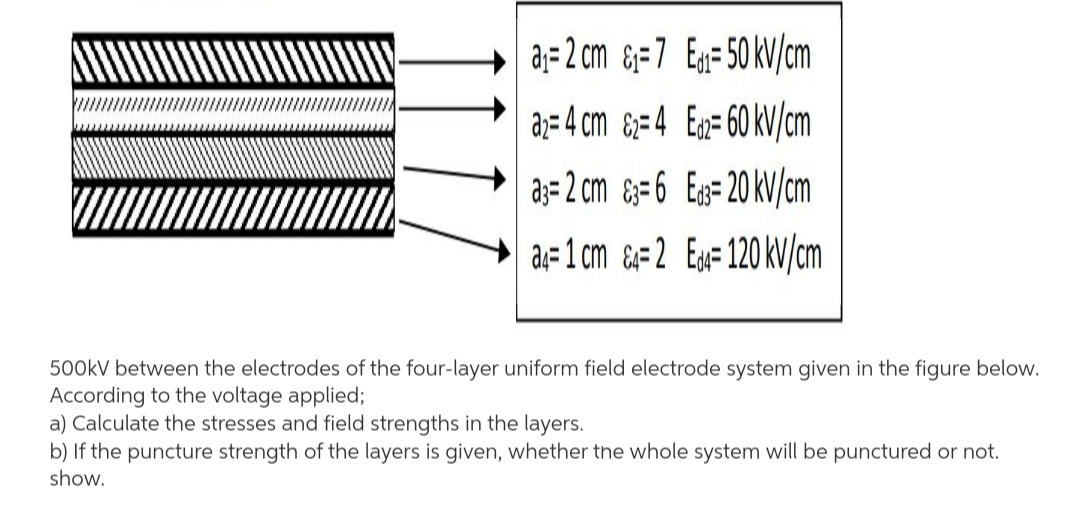# aj= 2 cm &=7 Eg=50 kV/cm a;= 4 cm &;=4 Eg= 60 kV/cm a;= 2 cm &=6 Eg=20 kV/cm a;=1 cm  Ex=120 kV/cm 500KV between the electrodes of the four-layer uniform field electrode system given in the figure below. According to the voltage applied; a) Calculate the stresses and field strengths in the layers.

Questionhelp_outlineImage Transcriptioncloseaj= 2 cm &=7 Eg=50 kV/cm a;= 4 cm &;=4 Eg= 60 kV/cm a;= 2 cm &=6 Eg=20 kV/cm a;=1 cm  Ex=120 kV/cm 500KV between the electrodes of the four-layer uniform field electrode system given in the figure below. According to the voltage applied; a) Calculate the stresses and field strengths in the layers. fullscreen

### Want to see this answer and more?

Experts are waiting 24/7 to provide step-by-step solutions in as fast as 30 minutes!*

*Response times may vary by subject and question complexity. Median response time is 34 minutes for paid subscribers and may be longer for promotional offers.
Tagged in
Engineering
Electrical Engineering

### Power Systems